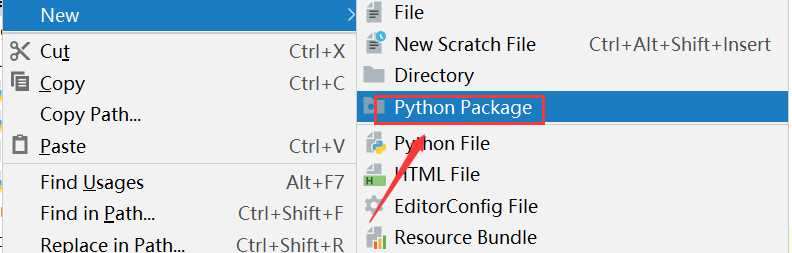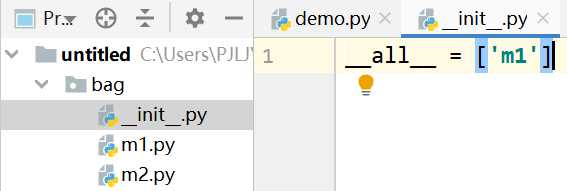# python基础入门之十八 —— 模块和包

## 一、模块

### 1、 导入模块

• import 模块名
• from 模块名 import 功能名
• from 模块名 import *
```# 法1：import 模块
import math
print(math.sqrt(9))```
```# 法2：from 模块 import 功能1，功能2，……
from math import sqrt
print(sqrt(8))```
```# 法3：from 模块 import *
from math import *
print(sqrt(8))```

### 2、模块别名

• import 模块名 as 别名
• from 模块名 import 功能名as 别名
```# 别名1：
import time as t
t.sleep(1)
# 别名2:
from time import sleep as sl
sl(1)```

### 3、制作模块

• __all__：在使用from 模块/包 import *时，允许导入的模块或功能列表
• __name__:运行该模块的文件名：（1）如果在本文件内运行__name__值为__main__
•                                                          (2)  如果在被其他文件调用时，__name__值为代码所在的文件名

```__all__ = [‘println‘,‘test‘]

def println(a,b):
print(a+b)

def test(a,b):
print(a*b)

if __name__ == ‘__main__‘:
println(1,2)
print(__name__)```

```from module import *

println(2,3)  # 5
test(1,2)  # 2```

P.s:如果功能名字重复，调用到的是最后定义或导入的功能

## 二 、包

• 将有联系的模块放在同一个文件夹下，并且在这个文件夹创建一个名字为__init__.py文件，那么这个文件夹就称作为包
• 新建包mypackage

1、创建包新建包后，包内部会自动创建__init__.py文件，这个文件控制着包的导入行为

## 2、导包

• import 包名.模块名
• from 包 import *

P.s:使用‘from 包 import *’这个导包一定要在__init__.py文件中定义__all__，否则报错m1.py:

```def info():
print(‘my module 1‘)```

m2.py:

```def info():
print(‘my module 2‘)```

demo.py:

```# 法一：import 包名.模块名
import bag.m1
bag.m1.info()  # my module 1

# 法二：from 包名 import *
from bag import *
m1.info()  # my module 1
# m2.info() # __all__列表中没有m2,报错```